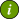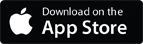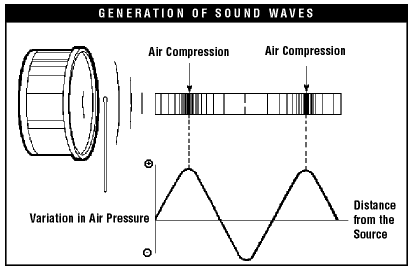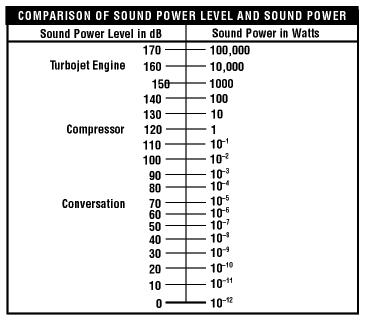### OSH Answers Fact SheetsEasy-to-read, question-and-answer fact sheets covering a wide range of workplace health and safety topics, from hazards to diseases to ergonomics to workplace promotion. MORE ABOUT >Search all fact sheets:

Type a word, a phrase, or ask a question

# Noise - Basic Information

## What are sound and noise?

Sound is what we hear. Noise is unwanted sound. The difference between sound and noise depends upon the listener and the circumstances. Rock music can be pleasurable sound to one person and an annoying noise to another. In either case, it can be hazardous to a person's hearing if the sound is loud and if they are exposed long and often enough.

Sound is produced by vibrating objects and reaches the listener's ears as waves in the air or other media. When an object vibrates, it causes slight changes in air pressure. These air pressure changes travel as waves through the air and produce sound. To illustrate, imagine striking a drum surface with a stick. The drum surface vibrates back and forth. As it moves forward, it pushes the air in contact with the surface. This creates a positive (higher) pressure by compressing the air. When the surface moves in the opposite direction, it creates a negative (lower) pressure by decompressing the air. Thus, as the drum surface vibrates, it creates alternating regions of higher and lower air pressure. These pressure variations travel through the air as sound waves (Figure 1).Figure 1

Table 1 lists the approximate velocity of sound in air and other media. In gases, the higher the velocity of sound, the higher the pitch will be (Remember the "Mickey Mouse" sound when people talk after inhaling helium gas?).

Table 1
Approximate Speed of Sound in Common Materials
Medium Sound
Velocity
(ft/s)
m/s
Air, dry (0°C and 760 mm Hg) 1,100 330
Wood (soft - along the fibre) 11,100 3,400
Water (15°C) 4,700 1,400
Concrete 10,200 3,100
Steel 16,000 5,000
Glass 18,500 5,500
Hydrogen (0°C and 760 mm Hg) 4,100 1,260

The hearing mechanism of the ear senses the sound waves and converts them into information which it relays to the brain. The brain interprets the information as sound. Even very loud sounds produce pressure fluctuations which are extremely small (1 in 10,000) compared to ambient air pressure (i.e., atmospheric pressure). The hearing mechanism in the ear is sensitive enough to detect even small pressure waves. It is also very delicate: this is why loud sound may damage hearing.

## Why is noise an important workplace hazard?

Noise is one of the most common occupational health hazards. In heavy industrial and manufacturing environments, as well as other situations such as farms, cafeterias, or bars. Permanent hearing loss is the main health concern. Annoyance, stress and interference with speech communication are the main concerns in noisy offices, schools and computer rooms.

To prevent adverse outcomes of noise exposure, noise levels should be reduced to acceptable levels. The best method of noise reduction is to use engineering modifications to the noise source itself, or to the workplace environment. Where technology cannot adequately control the problem, personal hearing protection (such as ear muffs or plugs) can be used. Personal protection, however, should be considered as an interim measure while other means of reducing workplace noise are being explored and implemented.

As a first step in dealing with noise, workplaces need to identify areas or operations where excessive exposure to noise occurs.

## How can I tell if my workplace is too loud?

If you answer yes to any of the following questions, the workplace may have a noise problem.

• Do people have to raise their voices?
• Do people who work in noisy environments have ringing in their ears at the end of a shift?
• Do they find when they return home from work that they have to increase the volume on their car radio higher than they did when they went to work?
• Does a person who has worked in a noisy workplace for years have problems understanding conversations at parties, or restaurants, or in crowds where there are many voices and "competing" noises?

If there is a noise problem in a workplace, then a noise assessment or survey should be undertaken to determine the sources of noise, the amount of noise, who is exposed and for how long.

## What are some properties of noise that can be measured?

The properties of noise which are important in the workplace are:

• frequency
• sound pressure
• sound power
• time distribution

## What are pitch and frequency?

Frequency is the rate at which the source produces sound waves, i.e. complete cycles of high and low pressure regions. In other words, frequency is the number of times per second that a vibrating body completes one cycle of motion. The unit for frequency is the hertz (Hz = 1 cycle per second: Figure 1 shows one cycle from an air compression to a minimum pressure and back to a maximum pressure).

The Harvard Dictionary of Music defines the pitch as a "stretch of sound whose frequency is clear and stable enough to be heard as not noise". The Oxford Dictionary defines pitch as "the quality of a sound governed by the rate of vibrations producing it; the degree of highness or lowness of a tone." Since the pitch is determined mostly by frequency, it is usually identified with it. The pitch depends also, in a lower degree, on the sound level and on the physiology of the auditory system.

Low pitched or bass sounds have low frequencies. High-pitched or treble sounds have high frequencies. A healthy, young person can hear sounds with frequencies from roughly 20 to 20,000 Hz. The sound of human speech is mainly in the range 300 to 3,000 Hz.

## What is sound pressure?

Sound pressure is the amount of air pressure fluctuation a noise source creates. We "hear" or perceive sound pressure as loudness. If the drum in our example (Figure 1) is hit very lightly, the surface moves only a very short distance and produces weak pressure fluctuations and a faint sound. If the drum is hit harder, its surface moves farther from its rest position. As a result, the pressure increase is greater. To the listener, the sound is louder.

Sound pressure also depends on the environment in which the source is located and the listener's distance from the source. The sound produced by the drum is louder two metres from the drum if it is in a small bathroom, than if it is struck in the middle of a football field. Generally, the farther one moves from the drum, the quieter it sounds. Also if there are hard surfaces that can reflect the sound (e.g., walls in a room), the sound will feel louder than if you heard the same sound, from the same distance, in a wide-open field.

Sound pressure is usually expressed in units called pascals (Pa). A healthy, young person can hear sound pressures as low as 0.00002 Pa. A normal conversation produces a sound pressure of 0.02 Pa. A gasoline-powered lawn mower produces about 1 Pa. The sound is painfully loud at levels around 20 Pa. Thus the common sounds we hear have sound pressure over a wide range (0.00002 Pa - 20 Pa).

## What is a sound pressure level?

It is difficult to work with the broad range of common sounds pressures (0.00002 Pa - 20 Pa). To overcome this difficulty we use decibel (dB, or tenth (deci) of a Bel)). The decibel or dB scale is more convenient because it compresses the scale of numbers into a manageable range.

The decibel is named after Alexander Graham Bell, the Canadian pioneer of the telephone who took great personal interest in the problems of the Deaf or people with hearing loss.

Sound pressure converted to the decibel scale is called sound pressure level (Lp). Appendix A gives a detailed explanation of decibels and sound pressure levels. Figure 2 compares sound pressures in pascals and sound pressure levels in decibels (dB). The zero of the decibel scale (0 dB) is the sound pressure of 0.00002 Pa. This means that 0.00002 Pa is the reference sound pressure to which all other sound pressures are compared on the dB scale. This is the reason the decibels of sound are often indicated as dB re 0.00002 Pa.Figure 2

## What is sound power?

The sound power is the sound energy transferred per second from the noise source to the air. A noise source, such as a compressor or drum, has a given, constant sound power that does not change if the source is placed in a different environment.

Power is expressed in units called watts (W). An average whisper generates a sound power of 0.0000001 watts (0.1 microwatt (µW)), a truck horn 0.1 W, and a turbo jet engine 100,000 W.

Like sound pressure, sound power (in W) is usually expressed as sound power levels in dB. Appendix B provides examples of sound power level calculations.

Figure 3 relates sound power in watts to sound power level in decibels. Note that while the sound power goes from one trillionth of a watt to one hundred thousand watts, the equivalent sound power levels range from 0 to 170 dB.Figure 3

## What is the relation between sound pressure and sound power?

Because the sound power of a noise source is constant and specific, it can be used to calculate the expected sound pressure. The calculation requires detailed information about the noise source's environment. Usually a noise source with a lower sound power generates less sound pressure. For example, from the sound power of a compressor, one can calculate the expected sound pressure and sound pressure level at a certain location and distance. This information can be helpful in determining possible noise exposures and how they compare to the noise guidelines.

The manufacturer can often provide noise emission data such as sound pressure levels under standard conditions and the sound power of equipment. A number of international standards are available for labelling machines and equipment with their noise emission levels. In Canada, the CSA Standard Z107.58-15 Noise Emission Declarations for Machinery provides manufacturers with a means to determine and to create noise emission declarations for the machinery they produce. In addition, Health Canada has issued a notice to stakeholders recommending that this noise data be included in the technical sales literature and instructions for use of machinery intended for a workplace.

## What kinds of noise are there?

Noise can be continuous, variable, intermittent or impulsive depending on how it changes over time. Continuous noise is noise which remains constant and stable over a given time period. The noise of boilers in a power house is relatively constant and can therefore be classified as continuous.

Most manufacturing noise is variable or intermittent. Different operations or different noise sources cause the sound changes over time. Noise is intermittent if there is a mix of relatively quiet and noisy periods. Impulse or impact noise is a very short burst of loud noise which lasts for less than one second. Gun fire or the noise produced by punch presses are examples of such noise.

## What are A-weighted decibels?

The sensitivity of the human ear to sound depends on the frequency or pitch of the sound. People hear some frequencies better than others. If a person hears two sounds of the same sound pressure but different frequencies, one sound may appear louder than the other. This difference occurs because people hear high frequency noise much better than low frequency noise.

Noise measurement readings can be adjusted to correspond to this peculiarity of human hearing. An A-weighting filter which is built into the sound measuring instrument de-emphasizes low frequencies or pitches. Decibels measured using a sound meter equipped with this filter are A-weighted and are called dB(A). Legislation on workplace noise normally gives exposure limits in dB(A). Table 2 lists examples of typical noise levels.

A-weighting serves two important purposes:

1. gives a single number measure of noise level by integrating sound levels at all frequencies
2. gives a scale for noise level as experienced or perceived by the human ear
Table 2
Typical Noise Levels
Noise Source dB(A)
pneumatic chipper at 1 metre 115
hand-held circular saw at 1 metre 115
chainsaw, leaf blower, snowmobile 106-115
ATV, motorcycle 96-100
subway, shouted conversation 90-95
power lawn mower at 1 metre 92
diesel truck 50 km per hour at 20 metres 85
passenger car 60 km per hour at 20 metres 65
conversation at 1 metre 55
quiet room 40

## What are basic rules of working with decibel (dB) units?

The decibel [dB, and also dB(A)] is a logarithmic scale. For mathematical calculations using dB units, we must use logarithmic mathematics (see Appendix A). However, in our day-to-day work we do not need such calculations.

The use of dB unit makes it easy to deal with the workplace noise level data provided we use a set of simple rules as summarized in Table 3.

Table 3
Decibel (dB) basics
Change in dB Change in sound energy
3 dB increase Sound energy doubled
3 dB decrease Sound energy halved
10 dB increase Sound energy increased by factor of 10
10 dB decrease Sound energy decreased by factor of 10
20 dB increase Sound energy increased by factor of 100
20 dB decrease Sound energy decreased by factor of 100

## How are noise levels added?

Sound pressure levels in decibels (dB) or A-weighted decibels [dB(A)] are based on a logarithmic scale (see Appendix A). They cannot be added or subtracted in the usual arithmetical way. If one machine emits a sound level of 90 dB, and a second identical machine is placed beside the first, the combined sound level is 93 dB, not 180 dB.

Table 4 shows a simple way to add noise levels.

Table 4
Numerical difference between
two noise levels [dB(A)]
Amount to be added to the higher of the
two noise levels [dB or dB(A)]
0 3.0
0.1 - 0.9 2.5
1.0 - 2.4 2.0
2.4 - 4.0 1.5
4.1 - 6.0 1.0
6.1 - 10 0.5
10 0.0
Step 1: Determine the difference between the two noise levels and find the corresponding row in the left hand column.
Step 2: Find the number [dB or dB(A)] corresponding to this difference in the right hand column of the table.
Step 3: Add this number to the higher of the two decibel levels.

For instance, using the example of two machines each emitting a noise level of 90 dB:

• Step 1: The numerical difference between the two levels is 0 dB (90-90= 0), using the first row.
• Step 2: The number corresponding to this difference of 0, taken from the right hand column, is 3.
• Step 3: Add 3 to the highest level, in this case 90. Therefore, the resulting noise level is 93 dB.

When the difference between two noise levels is 10 dB(A) or more, the amount to be added to the higher noise level is zero. In such cases, no adjustment factor is needed because adding in the contribution of the lower in the total noise level makes no perceptible difference in what people can hear or measure. For example if your workplace noise level is 95 dB(A) and you add another machine that produces 80 dB(A) noise, the workplace noise level will still be 95dB(A).

### Appendix A - Sound Pressure Level Calculations

Sound pressure level in decibels is defined in the following way:

dB = 20 log (Sound Pressure/Reference Pressure)

The "log" or logarithm of a number is a mathematical manipulation of the number, based on multiples of 10. It is the exponent that indicates the power to which the number 10 is raised to produce a given number. For example, the logarithm of 10 is 1 since 10 is multiplied by itself only once to get 10. Similarly, the logarithm of 100 is 2 since 10 times 10 is 100. The logarithm of 1000 is 3 since 10 times 10 times 10 is 1000.

Therefore

log(1) = 0 Since 10 to the exponent 0 = 1,

log(10) = 1 since 10 to the exponent 1 = 10,

log(100) = 2 since 10 to the exponent 2 = 100,

log(1000) = 3 since 10 to the exponent 3 = 1000

The logarithm scale simply compresses the large span of numbers into a manageable range. In other words, the scale from 10 to 1000 is compressed, by using the logarithms, to a scale of 1 to 3.

The decibel scale for sound pressures uses as the reference pressure the lowest noise that the healthy young person can hear (0.00002 Pa). It divides all other sound pressures by this amount when calculating the decibel value. Sound pressures converted to the decibel scale are called sound pressure levels, abbreviated Lp. So, the sound pressure level of the quietest noise the healthy young person can hear is calculated in this way:

Lp = 20 log ( 0.00002/ 0.00002) = 20 log (1) = 20 X 0 = 0 dB

The sound pressure level or Lp in a very quiet room, where the sound pressure is 0.002 Pa, is calculated:

Lp = 20 log (0.002/ 0.00002) = 20 log (100) = 20 X 2 = 40 dB

The sound pressure level of a typical gasoline-powered lawn mower, which has a sound pressure of 1 Pa, is calculated

Lp = 20 log (1/0.00002) = 20 log (50 000) = 20 X 4.7 = 94 dB

### Appendix B - Sound Power Level Calculations

Sound power levels or Lw are determined by the following formula:

Lw = 10 log (Sound Power Level / Reference Power Level )

The reference power is one trillionth of a watt (0.000000000001 W). Therefore

Lw = 10 log (Sound Power Level / 0.000000000001)

Thus, the sound power level associated with an average whisper, which has a sound power of 0.0000001 W, is calculated

Lw = 10 log (0.0000001/ 0.000000000001) = 50 dB

Document last updated on November 26, 2019